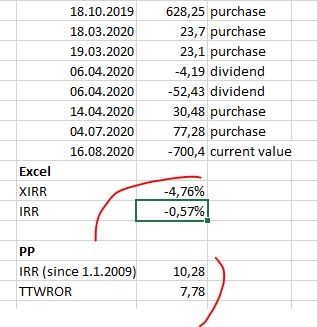# IRR/TTWROR calculation in PP vs. Excel

Hi

I was usin XIRR to calclate money-weighted returns for the portfolio and instruments. Moved to the software and decided ot check, whether the PP calculation of TTWROR and IRR correspond to the values that I would have expected to see.

Unfortunately, I recieved quite different results based on a test with one stock: PP reruts gives me positive values, although both Excle’s XIRR and IRR calculations give negativesWhat am I missing here? How can PP calculations be so much off?

How did you do the calculation in PP? Are you sure that no other transactions were included? Ideally, you could upload a minimal example PP file.

@Sunna

Did you really check your Excel calculation? Are the negative numbers correct?
As @chirlu asked you already rightly, are you sure that no other trades are incuded in the PP file? The number of trades and the purchase amounts in the Excel calculation have to be included in the same way in your PP file! I am sure you’ll find your mistake.

@Sunna

This evening I checked your Excel calculation with all numbers in your example and I came to the same results as you did using the Excel formulas. However, I found out that the total sum of all transactions from 18.10.2019 to 4.7.2020 add up to 726,19 and not to 700,4. So your investment lost around 26 of value (726,19 - 700 = value on Aug. 16, assuming that the value of 700,4 is correct), probably due to a loss in value.
It only proves that the negative results in Excel are correct.
Since PP shows positive results, there must be one or several mistakes in your data input in PP.

Thank you very much for the reply! And you are correct - there has been a loss in value. I rechecked the data, there was a small mistake with the number of shares in the Excel calculation. Basically what I did now is just input all PP transactions (same dates, same values) into Excel for XIRR,

With the current example even with same data I get a difference of 0.5% between Excel’s XIRR and PP’s IRR.

I did a similar check-up with 2 other stock. One (with just 4 transactions) gave me the same XIRR-IRR and the other had a difference of 0,09%.

It would really be interesting to understand what could be the error on the portfolio total level.